InfoQ Homepage Articles Fraud Detection Using Random Forest, Neural Autoencoder, and Isolation Forest Techniques

# Fraud Detection Using Random Forest, Neural Autoencoder, and Isolation Forest Techniques

Leia em Português

### Key Takeaways

• Fraud detection techniques mostly stem from the anomaly detection branch of data science.
• If the dataset has sufficient number of fraud examples, supervised machine learning algorithms for classification like random forest, logistic regression can be used for fraud detection.
• If the dataset has no fraud examples, we can use either the outlier detection approach using isolation forest technique or anomaly detection using the neural autoencoder.
• After the machine learning model has been trained, it's evaluated on the test set using metrics such as sensitivity and specificity, or Cohen’s Kappa.

With global credit card fraud loss on the rise, it is important for banks, as well as e-commerce companies, to be able to detect fraudulent transactions (before they are completed).

According to the Nilson Report, a publication covering the card and mobile payment industry, global card fraud losses amounted to \$22.8 billion in 2016, an increase of 4.4% over 2015. This confirms the importance of the early detection of fraud in credit card transactions.

Fraud detection in credit card transactions is a very wide and complex field. Over the years, a number of techniques have been proposed, mostly stemming from the anomaly detection branch of data science. That said, most of these techniques can be reduced to two main scenarios depending on the available dataset:

• Scenario  1: The dataset has a sufficient number of fraud examples.
• Scenario  2: The dataset has no (or just a negligible number of) fraud examples.

In the first scenario, we can deal with the problem of fraud detection by using classic machine learning or statistics-based techniques. We can train a machine learning model or calculate some probabilities for the two classes (legitimate transactions and fraudulent transactions) and apply the model to new transactions so as to estimate their legitimacy. All supervised machine learning algorithms for classification problems work here, e.g., random forest, logistic regression, etc.

In the second scenario, we have no examples for fraudulent transactions, so we need to get a bit more creative. Since all we have are examples of legitimate transactions, we need to make them suffice. There are two options for that: We can treat fraud as an outlier or as an anomaly and use a consistent approach. An example for the outlier detection approach is the isolation forest. A classic example for anomaly detection is the neural autoencoder.

Let’s take a look at how the different techniques can be used in practice on a real dataset. We implemented them on the fraud detection dataset from Kaggle. This dataset contains 284,807 credit card transactions, which were performed in September 2013 by European cardholders. Each transaction is represented by:

• 28 principal components extracted from the original data
• the time from the first transaction in the dataset
• the amount of money

The transactions have two labels: 1 for fraudulent and 0 for legitimate (normal) transactions. Only 492 (0.2%) transactions in the dataset are fraudulent, which is not really that many, but it may still be enough for some supervised training.

Notice that the data contain principal components instead of the original transaction features, for privacy reasons.

## Scenario 1: Supervised Machine Learning: Random Forest

Let’s start with the first scenario where we assume that a labeled dataset is available to train a supervised machine learning algorithm on a classification problem. Here we can follow the classical steps of a data science project: data preparation, model training, evaluation and optimization, and, finally, deployment.

### Data Preparation

Data preparation usually involves:

• Missing value imputation, if required by the upcoming machine learning algorithm
• Feature selection for improved final performance
• Additional data transformations to comply with the most recent regulations on data privacy

However, in this case, the dataset we chose has already been cleaned, and it is ready to use; no additional data preparation is needed.

All supervised classification algorithms need a training set to train the model and a test set to evaluate the model quality. After reading, the data, therefore, have to be partitioned into a training set and a test set. Common partitioning proportions vary between 80-20% and 60-40%. For our example, we adopted 70-30% partitioning, where 70% of the original data is put into the training set, and the remaining 30% is reserved as the test set for the final model evaluation.

For classification problems like the one at hand, we need to ensure that both classes — in our case, fraudulent and legitimate transactions — are present in the training and test sets. Since one class is much less frequent than the other, stratified sampling is advised here rather than random sampling. Indeed, while random sampling might miss the samples from the least numerous class, stratified sampling guarantees that both classes are represented in the final subset according to the original class distribution.

### Model Training

Any supervised machine learning algorithm could work. For demonstration purposes, we have chosen a random forest with 100 trees, all trained up to a depth of ten levels and with a maximum of three samples per node, using the information gain ratio as a quality measure for the split criterion.

### Model Evaluation: Making an Informed Decision

After the model has been trained, it has to be evaluated on the test set. Classic evaluation metrics can be used, such as sensitivity and specificity, or Cohen’s Kappa. All of these measures rely on the predictions provided by the model. In most data analytics tools, model predictions are produced based on the class with the highest probability, which in a binary classification problem is equivalent to using a default 0.5 threshold on one of the class probabilities.

However, in the case of fraud detection, we might want to be more conservative regarding fraudulent transactions. This means we would rather double-check a legitimate transaction and risk bothering the customer with a potentially useless call rather than miss out on a fraudulent transaction. In this case, the threshold of acceptance for the fraudulent class is lowered — or alternatively, the threshold of acceptance for the legitimate class is increased. For this case study, we adopted a decision threshold of 0.3 on the probability of the fraudulent class and compared the results with what we obtained with the default threshold of 0.5.

Figure 1 below shows the confusion matrices obtained using a decision threshold of 0.5 (on the left) and 0.3 (on the right), leading respectively to Cohen’s Kappa of 0.890 and 0.898 on an undersampled dataset with the same number of legitimate and fraudulent transactions. As you can see from the confusion matrices, privileging the decision toward fraudulent transactions produces a few additional legitimate transactions mistaken as fraudulent as the price to pay for more fraudulent transactions correctly identified.

[Click on the image to enlarge it]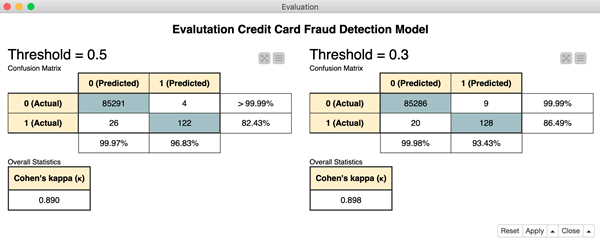Figure 1. The figure shows the performance measure of the random forest using two different thresholds: 0.5 on the left and 0.3 on the right. In the confusion matrix, class 0 refers to the legitimate transactions and class 1 to the fraudulent transactions. The confusion matrices show six more fraudulent transactions correctly classified using a lower threshold of 0.3.

### Hyperparameter Optimization

To complete the training cycle, the model parameters could be optimized — as for all classification solutions. We have omitted this part in this case study, but it could easily be introduced. For a random forest, this means finding the optimal number of trees and tree depth for the best classification performance (D. Goldmann, "Stuck in the Nine Circles of Hell? Try Parameter Optimization and a Cup of Tea," KNIME Blog, 2018; Hyperparameter optimization). In addition, the prediction threshold could also be optimized.

The workflow we used for training is therefore a very simple one with just a few nodes (Fig. 2): reading, partitioning, random forest training, random forest prediction generation, threshold application, and performance scoring. The workflow "Fraud Detection: Model Training" is available for free and can be downloaded from the KNIME Hub. To run the example simply download and install the open source tool KNIME Analytics Platform for free.

[Click on the image to enlarge it]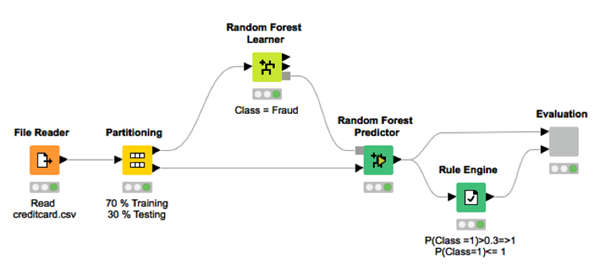Fig. 2. This workflow reads the dataset and partitions it into a training and a test set. Next, it uses the training set to train a random forest, applies the trained model to the test set, and evaluates the model performance for the thresholds 0.3 and 0.5.

### Deployment

Finally, when the model performance is acceptable by our standards, we can use it in production on real-world data.

The deployment workflow (Fig. 3) imports the trained model, reads one new transaction at a time, and applies the model to the input transaction and the custom threshold to the final prediction. In the event that a transaction is classified as fraudulent, an email is sent to the credit card owner to confirm the transaction’s legitimacy.

[Click on the image to enlarge it]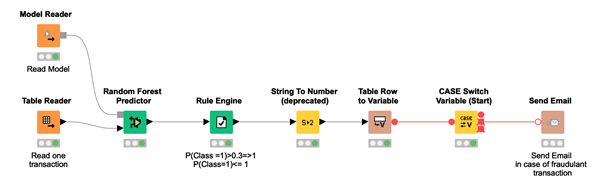Fig. 3. The deployment workflow reads the trained model, one new transaction at a time. It then applies the model to the input data, the defined threshold to the prediction probabilities, and sends an email to the credit card owner in case a transaction has been classified as fraudulent.

## Scenario 2: Anomaly Detection using Autoencoder

Let’s now move on to scenario 2. The fraudulent transactions in the dataset were so few anyway that they could simply be reserved for testing and completely omitted from the training phase.

One of the approaches that we have proposed stems from anomaly detection techniques. Anomaly detection techniques are often used to detect any exceptional or unexpected event in the data, be it a mechanical piece failure in IoT, an arrhythmic heartbeat in the ECG signal, or a fraudulent transaction in the credit card business. The complex part of anomaly detection is the absence of training examples for the anomaly class.

A frequently used anomaly detection technique is the neural autoencoder: a neural architecture that can be trained on only one class of events and used in deployment to warn us against unexpected new events. We will describe its implementation here as an example for the anomaly detection techniques.

### The Autoencoder Neural Architecture

As shown in Figure 4, the autoencoder is a feed-forward backpropagation-trained neural network with as many n input units as n output units. In the middle, it has one or more hidden layers with a central bottleneck layer with h units, where h < n. The idea here is to train the neural network to reproduce the input vector x to the output vector x'.

The autoencoder is trained using only examples from one of the two classes, in this case the class of legitimate transactions. During deployment, the autoencoder will therefore perform a reasonable job in reproducing the input x on the output layer x' when presented with a legitimate transaction and a less than optimal job when presented with a fraudulent transaction (i.e., an anomaly). This difference between x and x' can be quantified via a distance measure, e.g.,

$$d(x,x'):=\frac{1}{n}\displaystyle\sum_{i=1}^{n}(x_i-x'_i)^2$$

The final decision on legitimate transaction vs. fraudulent transaction is taken using a threshold value δ on the distance d(x,x'). A transaction x is a fraud candidate according to the following anomaly detection rule:

$$x →\quad "normal" IF \quad d(x,x') ≤ δ$$

$$x →\quad "fraud" IF \quad d(x,x')>δ.$$

[Click on the image to enlarge it]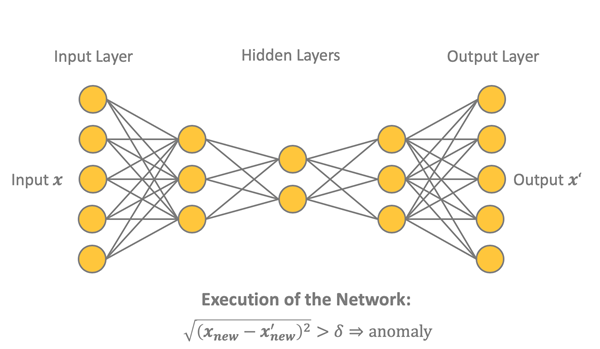Fig. 4. This figure shows a possible network structure for an autoencoder. In this case, we have five input units and three hidden layers with three, two and three units respectively. The reconstructed output x’ has again five units like the input. The distance between the input x and the output x’ can be used to detect anomalies, i.e., the fraudulent transactions.

The threshold value δ can, of course, be set conservatively to fire an alarm only for the most obvious cases of fraud or can be set less conservatively to be more sensitive toward anything out of the ordinary. Let’s see the different phases involved in this process.

### Data Preparation

The first step in this case is to isolate a subset of legitimate transactions to create the training set in order to train the network. Of all legitimate transactions in the original dataset, 90% of them were used to train and evaluate the autoencoder network and the remaining 10%, together with the remaining fraudulent transactions, to build the test set for the evaluation of the whole strategy.

The usual data preparation steps should apply to the training set, as we discussed above. However, as we have also seen before, this dataset has already been cleaned, and it is ready to be used. No additional classic data preparation steps are necessary; the only step we need to take is a neural network custom required step: normalization of the input vectors to fall in [0,1].

### Building and Training the Neural Autoencoder

The autoencoder network is defined as a 30-14-7-7-30 architecture, using tanh and ReLU activation functions and activity regularizer L1 = 0.0001, as suggested in the blog post "Credit Card Fraud Detection using Autoencoders in Keras — TensorFlow for Hackers (Part VII)" by Venelin Valkov. The activity regularization parameter L1 is a sparsity constraint, which makes the network less likely to overfit the training data.

The network is trained until the final loss values are in the range [0.070, 0.071], according to the loss function mean squared error (MSE):

$$MSE=\frac{1}{n}\displaystyle\sum_{i=1}^{N}\displaystyle\sum_{j=1}^{n}(x'_{ij}-x_{ij})^2 ,$$

where N is the batch size and n is the number of units on the output and input layer.

The number of training epochs is set to 50, the batch size N is also set to 50, and Adam — an optimized version of the backpropagation algorithm — is chosen as the training algorithm. After training, the network is saved for deployment as a Keras file.

### Model Evaluation: Making an Informed Decision

The value of the loss function, however, does not tell the whole story. It just tells us how well the network is able to reproduce "normal" input data onto the output layer. To have a full picture of how well this approach performs in detecting fraudulent transactions, we need to apply the anomaly detection rule mentioned above to the test data, including the few frauds.

In order to do this, we need to define the threshold δ for the fraud alert rule. A good starting point for the threshold comes from the final value of the loss function at the end of the learning phase. We used δ = 0.009, but as mentioned earlier, this is a parameter that could be adapted depending on how conservative we want our network to be.

Figure 5 shows the network and distance-based rule performance on the test set made of 10% of the legitimate transactions and all of the fraudulent transactions.

[Click o the image to enlarge it]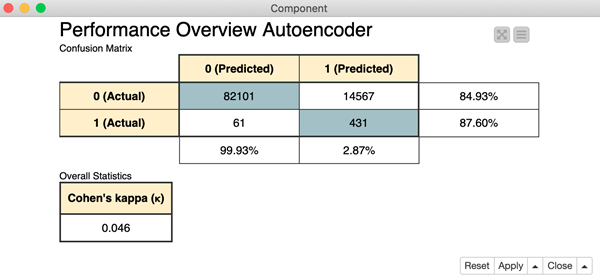Fig. 5. This figure gives a performance overview for the autoencoder model, including the confusion matrix and the Cohen’s Kappa. Again, 0 represents the class of legitimate transactions and 1 the class of fraudulent transactions.

The final workflow — building the autoencoder neural network, partitioning the data into training and test set, normalizing the data before feeding them into the network, training the network, applying the network to the test data, calculating the distance d(x,x'), applying the threshold δ, and finally scoring the results — is shown in figure 6 and is available for download on the KNIME Hub at Keras Autoencoder for Fraud Detection Training.

[Click on the image to enlarge it]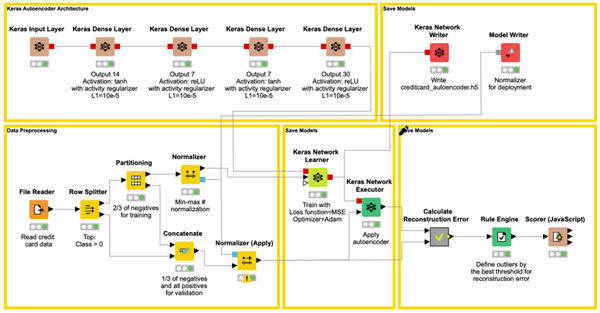Fig. 6. This workflow reads the credit card.csv dataset and creates a training set, using 90% of all legitimate transactions only, and a test set using the remaining 10% of legitimate transactions and all of the fraudulent transactions. The autoencoder network is defined in the upper left part of the workflow. After data normalization, the autoencoder is trained and its performance is evaluated.

### Deployment

Now we have reached the deployment phase. In the deployment application, the trained autoencoder is read and applied to the new normalized incoming data, the distance between input vector and output vector is calculated, and the threshold is applied. If the distance is below the threshold, the incoming transaction is classified as legitimate, otherwise as fraudulent.

Notice that the network plus threshold strategy has been deployed within a REST application, accepting input data from the REST Request and producing the predictions in the REST Response.

The workflow implementing the deployment is shown in figure 7 and can be downloaded from the KNIME Hub at Keras Autoencoder for Fraud Detection Deployment.

[Click on the image to enlarge it]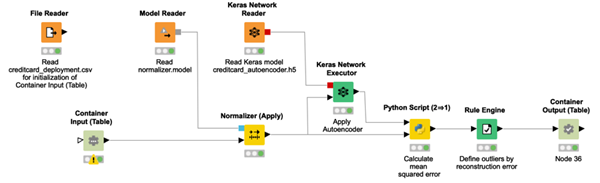Fig. 7. The execution of this workflow can be triggered via REST from any application by sending a new transaction in the REST Request structure. The workflow then reads and applies the model to the incoming data and sends back the corresponding prediction, either 0 for legitimate or 1 for fraudulent transaction.

## Outlier Detection: Isolation Forest

Another group of strategies for fraud detection — in the absence of enough fraud examples — relies on techniques for outlier detection. Among all of the many available outlier detection techniques, we propose the Isolation Forest technique (M. Widmann and M. Heine, "Four Techniques for Outlier Detection," KNIME Blog, 2019).

The basic idea of the Isolation Forest algorithm is that an outlier can be isolated with less random splits than a sample belonging to a regular class, as outliers are less frequent than regular observations and have values outside of the dataset statistics.

Following this idea, the Isolation Forest algorithm randomly selects a feature and randomly selects a value in the range of this feature as the split value. Using this partitioning step recursively generates a tree. The number of required random splits (the isolation number) to isolate a sample is the tree depth. The isolation number (often also called the mean length), averaged over a forest of such random trees, is a measure of normality and our decision function to identify outliers. Random partitioning produces noticeably shorter tree depths for outliers and longer tree depths for other data samples. Hence, when a forest of random trees collectively produces shorter path lengths for a particular data point, this is likely to be an outlier.

### Data Preparation

Again, the data preparation steps are the same as mentioned above: missing value imputation, feature selection, and additional data transformations to comply with the most recent regulations on data privacy. As this dataset has already been cleaned, it is ready to be used. No additional classic data preparation steps are necessary. The training and test sets are created in the same way as in the autoencoder example.

### Training and Applying Isolation Forest

Thus, an Isolation Forest with 100 trees and a maximum tree depth of eight is trained, and the average isolation number for each transaction across the trees in the forest is calculated.

### Model Evaluation: Making an Informed Decision

Remember, the average isolation number for outliers is smaller than for other data points. We adopted a decision threshold δ=6. Therefore, a transaction is defined as a fraud candidate if the average isolation number lies below that threshold. As in the other two examples, this threshold is a parameter that can be optimized, depending on how sensitive we want the model to be.

Performances for this approach on the test set are shown in Fig. 8. The final workflow, available on the KNIME Hub here, is shown in Fig. 9.

[Click on the image to enlarge it]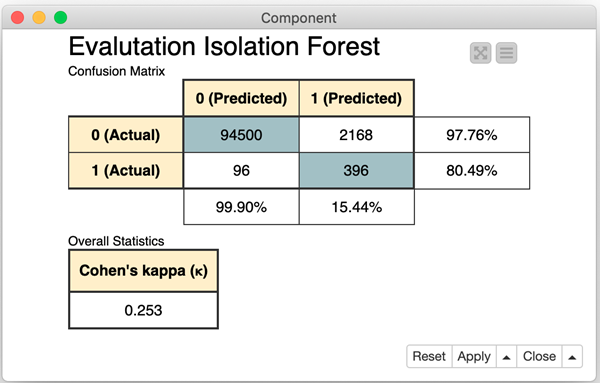Fig. 8. Performance measures for the Isolation Forest on the same test set as for the autoencoder solution, including the confusion matrix and the Cohen’ Kappa. Again, 0 represents the class of legitimate transactions and 1 the class of fraudulent transactions.

[Click on the image to enlarge it]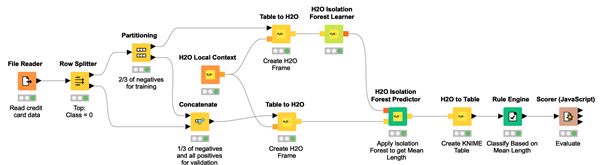Fig. 9. The workflow reads the credit card.csv dataset, creates the training and test sets, and transforms them into H2O Frame. Next, it trains an Isolation Forest and applies the trained model to the test set to find outliers based on the isolation number of each transaction.

### Deployment

The deployment application here reads the Isolation Forest model and applies it to the new incoming data. Based on the threshold defined during training and applied to the isolation number value, the incoming data point is identified as either a candidate fraud transaction or a legitimate transaction.

[Click on the image to enlarge it]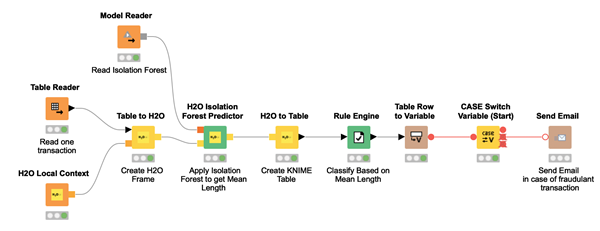Fig. 10. The deployment workflow reads a new transaction and applies the trained Isolation Forest to this new transaction. The isolation number is calculated for each input transaction and decides whether a transaction is fraudulent. In case of fraudulent transactions, the workflow sends an email to the owner of the credit card.

## Summary

As described at the beginning of this tutorial, fraud detection is a wide area of investigation in the field of data science. We have portrayed two possible scenarios depending on the available dataset: a dataset with data points for both classes of legitimate and fraudulent transactions and a dataset with either no examples or only a negligible number of examples for the fraud class.

For the first scenario, we suggested a classic approach based on a supervised machine learning algorithm, following all the classic steps in a data science project as described in the CRISP-DM process. This is the recommended way to proceed. In this case study, we implemented an example based on a random forest classifier.

Sometimes, due to the nature of the problem, no example for the class of fraudulent transactions is available. In these cases, less accurate approaches which are nevertheless still feasible become appealing. For this second scenario, we have described two different approaches: the neural autoencoder from the anomaly detection techniques and the Isolation Forest from the outlier detection techniques. As in our example, often both of them are not as accurate as the random forest, but in some cases, we have no other possible approach to use.

The three approaches proposed here are surely not the only ones that can be found in literature. However, we believe that they are representative of the three commonly used groups of solutions for the fraud detection problem.

Notice that the last two approaches were discussed for cases in which labeled fraud transactions are not available. These are kind of emergency approaches, to be used when the classic approach for classification cannot be applied for lack of labeled data in the fraud class. We recommend using a supervised classification algorithm whenever possible. However, when no fraud data is available, one of the last two approaches could be of help. Indeed, while being prone to produce false positives, they are in some cases the only possible ways to deal with the problem of fraud detection.Kathrin Melcher is a data scientist at KNIME. She holds a master’s degree in mathematics from the University of Konstanz, Germany. She enjoys teaching and applying her knowledge to data science, machine learning and algorithms. Follow Kathrin on LinkedIn.Rosaria Silipo, Ph.D., principal data scientist at KNIME, is the author of 50+ technical publications, including her most recent book "Practicing Data Science: A Collection of Case Studies." She holds a doctorate degree in bio-engineering and has spent more than 25 years working on data science projects for companies in a broad range of fields, including IoT, customer intelligence, the financial industry, and cybersecurity. Follow Rosaria on Twitter and LinkedIn.

Style

## Hello stranger!

You need to Register an InfoQ account or or login to post comments. But there's so much more behind being registered.

Get the most out of the InfoQ experience.

Allowed html: a,b,br,blockquote,i,li,pre,u,ul,p

Allowed html: a,b,br,blockquote,i,li,pre,u,ul,p

Allowed html: a,b,br,blockquote,i,li,pre,u,ul,p

Is your profile up-to-date? Please take a moment to review and update.

Note: If updating/changing your email, a validation request will be sent

Company name:
Company role:
Company size:
Country/Zone:
State/Province/Region:
You will be sent an email to validate the new email address. This pop-up will close itself in a few moments.